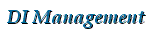DI Management Home > Mathematics > RSA: how to factorize N given d

#RSA: how to factorize N given d

This page explains how to factorize the RSA modulus $N$ given the public and private exponents, $e$ and $d$.

## Introduction

For the RSA algorithm, we have a public key $(N, e)$ and a private key $(N, d)$ where $N = pq$ is the product of two distinct primes $p$ and $q$, and the numbers $e$ and $d$ satisfy the relation $ed \equiv 1 \mod \phi(N)$ where $\phi(N) = (p-1)(q-1)$. $N$ should be a large number which is impossible to factorize, typically of length 1024 bits. The numbers $N$ and $e$ can be made public, but $d$, $p$, $q$ and $\phi(N)$ are kept secret by the user of the private key. See our RSA Algorithm and RSA Theory pages for more information.

## The problem: given d and e, can we factorize N?

Surprisingly, there isn't a simple formula to compute the factors $p$ and $q$ of the modulus $N$ given just the public and private exponents, $e$ and $d$. But there is a nice efficient algorithm using a random $g$ which should succeed about half the time.

Initially we compute $k = de-1$. We then choose a random integer $g$ in the range $1 \lt g \lt N$. Now $k$ is an even number, where $k = 2^tr$ with $r$ odd and $t\geq1$, so we can compute $x = g^{k/2}, g^{k/4}, \ldots, g^{k/2^t} \pmod N$ until $x \gt 1$ and $y = \gcd(x-1, N) \gt 1$. If so, then one of our factors, say $p$, is equal to $y$, and the other is $q=N/y$ and we are done. If we don't find a solution, then we choose another random $g$.

Algorithm
Input: $N$, $e$, $d$.
Output: $p$ and $q$ where $pq = N$.
1. [Initialize] Set $k \leftarrow de - 1$.
2. [Try a random g] Choose $g$ at random from $\{2,\ldots,N-1\}$ and set $t \leftarrow k$.
3. [Next t] If $t$ is divisible by $2$, set $t \leftarrow t/2$ and $x \leftarrow g^t \mod N$. Otherwise go to step 2.
4. [Finished?] If $x \gt 1$ and $y = \gcd(x-1, N) \gt 1$ then set $p \leftarrow y$ and $q \leftarrow N/y$, output $(p,q)$ and terminate the algorithm. Otherwise go to step 3.

## A simple example

Input: $N=25777$, $e=3$, $d=16971$.

k=de-1=50912

Trying g=2
t=25456
x=g^t mod N=1
t=12728
x=g^t mod N=1
t=6364
x=g^t mod N=1
t=3182
x=g^t mod N=25776
y=gcd(x-1,N)=1
t=1591
x=g^t mod N=12709
y=gcd(x-1,N)=1

Trying g=5
t=25456
x=g^t mod N=1
t=12728
x=g^t mod N=1
t=6364
x=g^t mod N=1
t=3182
x=g^t mod N=15050
y=gcd(x-1,N)=149
p=149
q=N/p=25777/149=173

Output: p=173, q=149


Note that we swapped $p$ and $q$ here in accordance with the convention that $p \gt q$.

## Code to do this with large integers

We use our BigDigits multiple-precision arithmetic software to implement this algorithm for large integers. The code is here. Note that we cheat slightly by just choosing small primes $g=2,3,5,7,11,\ldots$ instead of random values for $g$. We should get a result within a few tries.

The output for the 508-bit example from [KALI93] should be as follows:

Input:
n=a66791dc6988168de7ab77419bb7fb0c001c62710270075142942e19a8d8c51d053b3e3782a1de
5dc5af4ebe99468170114a1dfe67cdc9a9af55d655620bbab
e=10001
d8bd50e94bfc723fa87d8862b75177691c11d757692df8881
k=de-1=3912086784511471289561151952301925086168946454709316095960618800742799369
17336572480276788289479037864022477149770074463282234977724373626952267297821665
0880
Trying g=2
k1=19560433922557356447805759761509625430844732273546580479803094003713996845866
82862401383941447395189320112385748850372316411174888621868134761336489108325440
x=g^{k1} mod N=1
k1=97802169612786782239028798807548127154223661367732902399015470018569984229334
1431200691970723697594660056192874425186158205587444310934067380668244554162720
x=g^{k1} mod N=1
k1=48901084806393391119514399403774063577111830683866451199507735009284992114667
0715600345985361848797330028096437212593079102793722155467033690334122277081360
x=g^{k1} mod N=1
k1=24450542403196695559757199701887031788555915341933225599753867504642496057333
5357800172992680924398665014048218606296539551396861077733516845167061138540680
x=g^{k1} mod N=15093433718268440426031307226229967304531280096624631832802133467
75503932569655700680786404281006639009247399377287658652217161876478659236009863
49707225
y=gcd(x-1,N)=2323495016218899338815592763008533131685106005533447038236880433183
4850828939
Output:
p=33d48445c859e52340de704bcdda065fbb4058d740bd1d67d29e9c146c11cf61
q=335e8408866b0fd38dc7002d3f972c67389a65d5d8306566d5c4f2a5aa52628b


which is indeed the correct factorization.